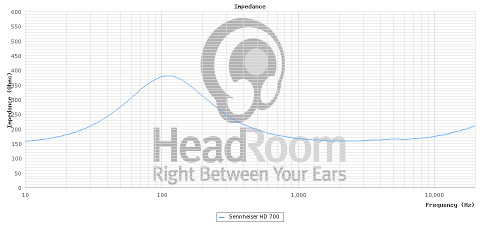Discussion in 'Sound Science' started by proton007, Apr 25, 2012.

1.

Is it possible to generate the THD plots in dBV instead of %?

2.
So ... it can not be calculated this way ->

?

Found out how "5.5V into 150 ohms = 200 mW max." works -> "P = V2/ R" is it right formula ?

Than the correct output power would be "72/150=326.6 mW" ?

Also what about max impedance ? Nominal is actually only lowest impedance.

So shouldn't we calculate with max impedance ?This graph shows 370ohm@120Hz so "72/370=132.4 mW"  would be more correct ?

Lastly I think we still got something wrong.

There are specs for some amp with different ohms already calculated.

Max. output level:
> 18.8 Veff in 600 ohms = 590 mW
> 13.8 Veff in 100 ohms = 1900 mW
> 10.7 Veff in 50 ohms = 2300 mW
> 7.8 Veff in 50 ohms = 1900 mW
> 3.7 Veff in 16 ohms = 410 mW

Veff should equal Vp-p if I didn't get it wrong and when I do calculations nearest number I got was 540mW but no luck with 590When used formula "V=Vp-p/2.8284" and "P=V2/R" I end up absolutely off.

3. Two things;
Pavg = Average power, calculated using Vrms
Pp-p = Max possible power,  calculated using Vp-p.

In the lake people specs, V-effective means Vrms.

Most of the times, Vrms is the unit used in AC specs.

4.
Ah, now it works "18.82/600=590"

5.
Yes. I used % because I thought it is a more familiar distortion unit for most people. I still have the recorded WAV files, though, so I can generate more graphs (including separate D2 and D3) if needed.

6.
You should generally use the nominal impedance, since that is approximately the impedance at 1 kHz, and the sensitivity of the headphones is typically also specified at 1 kHz. An amplifier with very low output impedance is basically a voltage source (as long as it can supply the required current), so it does not need to output a higher voltage at 120 Hz than at 1 kHz, otherwise it would not have a flat frequency response. You can view the increased impedance at 120 Hz as increased efficiency, due to the fact that the driver resonates at that frequency (requiring less power to produce the same SPL).

7.
Makes sense. It's just a thought.

The reason I asked is because I think % is relative to fundamental. For the headphone and microphone measurements, the fundamentals with the different series resistors have the same level, so % distortion are directly comparable. For the amp out measurement however, the fundamentals have different levels.

It might also be helpful to see all plots in dBV to compare distortion of headphone driver and amp out relative to a reference voltage instead of the different fundamentals.

8. My head wants to explode reading the stuff here. So if you don't mind I'll ask a question directly. Is the fiio e11 amp a good enough match with the grado sr80i? my source is sansa fuze+. I'm on a tight budget for the amp so the fiio e11 is all i can afford to get if ever. Thank you.

9. Hi sound science,

I've been trying to get my head around damping. So i decided to make a spreadsheet to tell me how much source resistance increases the reverberation decay time of a signal at system resonance. I used a closed box model (is this ok for open headphones?) to get the Qtc of a system with and without source resistance. I estimated T-S parameters based on plots from inner fidelity, I know thats not very accurate but I was looking for ballpark figures for Qms and Qes. What I found so far was that using a dampening factor of 8 would increase the decay time by a few percent. I'm yet to convert the decay time to reference a more usable measure like a drop in db. Here's the spreadsheet if anyone wants to correct/look/play. Blue fields are inputs.

Neug

10. Hello, Neug.

First, the word you should be using is "damping," not "dampening." Damping refers to the control of the speaker's resonance which is what you're talking about here. Dampening refers to the absorption of sound inside the enclosure. For example, you would call the fiberglass or poly fill stuffing inside a speaker enclosure "dampening material."

Second, I'm not sure just what decay you're talking about. You use -60dB as reference, which to me sounds like you're talking about reverberation decay, i.e. RT60 which is used to describe the reverberation time within an acoustic space. Is that what you're referring to or something else?

se

Neug likes this.

11. Thanks for the corrections. Post edited. I'm not familiar with a lot of the terms of acoustics. Yes it's reverberation decay, exactly (mathematically).

Neug

12. So you're trying to figure out the reverberation decay of a pair of headphones? Presumably while they're being worn? I'm afraid that's not making any sense to me seeing as the acoustic space between the drivers and the wearer's head is incredibly small. Nor do I see how the speaker's Qtc would have any effect whatsoever on the RT60 of an acoustic space. That's a function of the acoustic space, not the driver or loudspeaker. Where did the RT60 figures in your spreadsheet come from?

se

13. I'm calculating 2.2*Qtc/fc. The speaker in a closed box has a Q and resonant frequency so it should have a decay time. Maybe reverberation time is the wrong word.

Cheers
Neug

14. Lecture on Decay of oscillations.

Neug

15. Ok, so basically what you're talking about is what would rather commonly be called ringing.

se